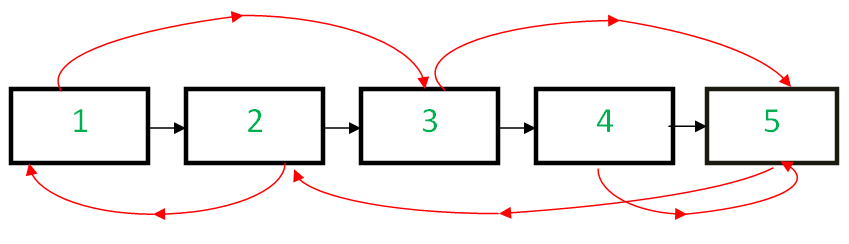# Copy List with Random Pointer

Posted: 13 Nov, 2020
Difficulty: Easy

## PROBLEM STATEMENT

#### For example,#### Random pointers are shown in red and next pointers in black.

##### Input Format:
``````The first line of input contains an integer ‘T’ representing the number of test cases. Then the test cases follow.

The only line of each test case contains the elements of the linked-list with random pointers. The line consists of nodes (value of node followed by its random pointer) separated by a single space. In case a node (next or random pointer) is null, we take -1 in its place.

Each node is represented as a pair of a value and its random index where,
Value: an integer representing the value of the node
Random index: the index of the node where the random pointer points to, or -1 if it does not point to any node.

For example, the input for the linked list depicted in the below image would be:
````````````1 2 2 0 3 4 4 4 5 1 -1
``````

#### Explanation:

``````The head node of the linked-list is 1, and its random pointer points to a node present at index 2, i.e. node 3.

The second node of the linked list is 2, and its random pointer points to a node present at index 0, i.e. node 1.

In this way, input for each node is taken until a pair having its first part as -1 is encountered since it denotes a node having null value, i.e. end of the linked list.
``````
##### Output Format:
``````For each test case, the only output line contains “true” if the linked list is successfully cloned.

The output for each test case is in a separate line.
``````
##### Note:
``````You do not need to print anything, it has already been taken care of. Just implement the given function.
``````
##### Constraints:
``````1 <= T <= 10 ^ 2
0 <= N <= 10 ^ 3
0 <= DATA <= 10 ^ 6 and data != -1
-1 <= RANDOMINDEX < N

Where ‘T’ is the number of test cases, ‘N’ is the total number of nodes in the linked list, 'DATA' is the value of the linked list node and 'RANDOMINDEX' is the index of the node where the random pointer points to.

Time limit: 1 sec.
``````
``````Can you do it without using extra space?
``````Approach 1

The basic idea behind the recursive solution is to consider the linked list like a binary tree. Every node of the Linked List has 2 pointers. So we consider these nodes as belonging to a binary tree. The head of the list becomes the root of the tree. So what we basically have to do now is to traverse the binary tree and clone it. The main issue that we need to tackle here is that of loops. So we can have, 1 1 2 0 -1, we need to handle this in the code.

We start from the root node, and we keep traversing the tree (list), and we keep generating new nodes whenever we find a node for which the clone has not been generated. For example, we were at node A, and we used the next pointer to go to node B, and we created B’, which is a new node B with the same data. Also, say there was a random pointer from A to B. In this case, we don’t have to create yet another copy of node B because B’ already exists. We need to take care of this as well.

Steps are as follows:

1. Base case: If the current node is not present in the linked list, return null.
2. Check if we have already processed the current node (using HashMap), then simply return the cloned version of it.
3. Else, create a new node with the data same as the old node, i.e. copy the node.
4. Save this value in the HashMap. This is needed to avoid the loops which might be there while traversing the list.
5. Recursively copy the remaining linked list starting once from the next pointer and then from the random pointer.
6. Finally, update the next and random pointers for the new node created and return it.